International
Tables for
Crystallography
Volume D
Physical properties of crystals
Edited by A. Authier

International Tables for Crystallography (2006). Vol. D, ch. 1.1, p. 5

## Section 1.1.1.5. Onsager relations

A. Authiera*

aInstitut de Minéralogie et de la Physique des Milieux Condensés, Bâtiment 7, 140 rue de Lourmel, 75015 Paris, France
Correspondence e-mail: aauthier@wanadoo.fr

#### 1.1.1.5. Onsager relations

| top | pdf |

Let us now consider systems that are in steady state and not in thermodynamic equilibrium. The intensive and extensive parameters are time dependent and relation (1.1.1.3)can be written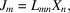where the intensive parameters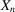are, for instance, a temperature gradient, a concentration gradient, a gradient of electric potential. The corresponding extensive parameters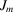are the heat flow, the diffusion of matter and the current density. The diagonal terms of matrix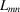correspond to thermal conductivity (Fourier's law), diffusion coefficients (Fick's law) and electric conductivity (Ohm's law), respectively. Non-diagonal terms correspond to cross effects such as the thermoelectric effect, thermal diffusion etc. All the properties corresponding to these examples are represented by tensors of rank 2. The case of second-rank axial tensors where the symmetrical part of the tensors changes sign on time reversal was discussed by Zheludev (1986).

The Onsager reciprocity relations (Onsager, 1931a,b)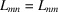express the symmetry of matrix. They are justified by considerations of statistical thermodynamics and are not as obvious as those expressing the symmetry of matrix (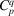). For instance, the symmetry of the tensor of rank 2 representing thermal conductivity is associated with the fact that a circulating flow is undetectable.

Transport properties are described in Chapter 1.8of this volume.

### References

Onsager, L. (1931a). Reciprocal relations in irreversible processes. I. Phys. Rev. 37, 405–426.
Onsager, L. (1931b). Reciprocal relations in irreversible processes. II. Phys. Rev. 38, 2265–2279.
Zheludev, I. S. (1986). Space and time inversion in physical crystallography. Acta Cryst. A42, 122–127.